Stained Glass Project

Stained Glass Linear Equations Project

DUE: ____________________________________

1) Choose 3 linear equations from each column (12 equations in total).

2) For each of the 12 equations, create a separate table.
3) State the slope and the y-intercept.
4) Graph all 12 equations on the coordinate plane provided. Use a ruler.
5) In BLACK pen, draw over the lines. Neatly write the equation on each line.
6) Use colored pencils or crayons to color in sections to create your stained glass window.

Category Score
Color, neatness,
and attractiveness
T-table
Y-intercepts and
coordinates
identified
Slope Identified
Graphing of linear
equations
Total
RUBRIC

Category 20 15 10 5
Color, Neatness, Exceptionally well Neat and Lines are neatly Appears messy
and Attractiveness designed, neat, and relatively drawn but graph and “thrown
attractive. Colors attractive. Ruler appears quite together” in a
that go well together was used to make plain. hurry. Lines are
are showed to make graphs more visibly crooked.
A ruler was used.
T-table The t-table is The t-table is The t-table is The t-table is not
included in the included in the partially included in the
project neatly project completed in the project.
numbered and project.
organized.
Y-intercept and Coordinates are Some coordinates Some coordinates Y-intercept is
Coordinates correctly plotted and are plotted are plotted identified.
Identified the y-intercepts are corrected and y- correctly.
named for each intercepts are
equation. identified.
Slope Identified The slope is The slopes are Some of the The slopes are not
identified as m identified slopes are identified
equals rise/run identified. correctly.
Graphing of The linear equations The equations are Some of the The equations are
Linear Equations are graphed correctly graphed correctly equations are graphed
and the coordinate and the coordinate graphed correctly incorrectly and
plane is labeled plane is labeled. and the coordinate coordinate plane
correctly. plane is labeled. is not labeled.

Circle 3 equations from each column.

𝑥 = −8 𝑦 = −9 𝑦 =𝑥+5 𝑦 = −𝑥 − 2

𝑥 = −5 𝑦 = −5 𝑦 = 2𝑥 − 7 𝑦 = −2𝑥 + 7

𝑥 = −1 𝑦 = −2 𝑦 = 4𝑥 + 1 𝑦 = −2𝑥

3
𝑥=2 𝑦=1 𝑦 = 𝑥+2 𝑦 = −𝑥 + 3
5
1 1
𝑥=7 𝑦=6 𝑦 = 𝑥−6 𝑦 = − 𝑥−4
4 3
1 3
𝑥=9 𝑦=8 𝑦 = 𝑥−3 𝑦 = − 𝑥+5
2 2
Complete one table for each equation you circled on the previous page.

x rule: y (x,y) x rule: y (x,y) x rule: y (x,y)

slope: _____ y-intercept: _____ slope: _____ y-intercept: _____ slope: _____ y-intercept: _____

x rule: y (x,y) x rule: y (x,y) x rule: y (x,y)

slope: _____ y-intercept: _____ slope: _____ y-intercept: _____ slope: _____ y-intercept: _____

x rule: y (x,y) x rule: y (x,y) x rule: y (x,y)

slope: _____ y-intercept: _____ slope: _____ y-intercept: _____ slope: _____ y-intercept: _____

x rule: y (x,y) x rule: y (x,y) x rule: y (x,y)

slope: _____ y-intercept: _____ slope: _____ y-intercept: _____ slope: _____ y-intercept: _____
STAINED GLASS WINDOW

Sours: https://es.scribd.com/document/393386930/Stained-Glass-Project

Assessment of Graphing Lines through Art!

Math can play an important role in art. In many works of art lines play a big role in the representation of figures, landscapes, and abstract works of imagination. This slide show from the NY Times shows several abstract works of art in which lines play a prominent role.

Materials needed for the Stained Glass Project:

• Large piece of graph paper- some students used regular size
• Coloring supplies- markers, colored pencils
• Rulers

After showing students the slide show from the NY Times, I ask students to read the Instructions for the Stained Glass Window Activity on their own. I also show the students the Feedback Form before they start the activity to go over the checkpoints of the exercise that I think will help them be more successful on this activity. After answering any questions that come up, I set them off to follow the instructions.

While students are working, I am walking around to monitor and to complete the teacher part of the Feedback Form for students early on in the activity. An important point of the Instructions that I reiterate to students throughout the activity is the importance of writing the equation for each of the lines used in their design. I allow students to work about 10 to 15 minutes before stopping the class to have students get some peer feedback on their Feedback Form. I find that this activity works better when my students receive feedback while the lines are in pencil and can be easily erased.

I expect most of my students will complete graphing the 12 required lines before leaving class.  Many will complete the coloring of their design for homework.

Sours: https://betterlesson.com/lesson/resource/2429981/stained-glass-window-project-pdf

Stained Glass Window Project Graphing Linear Equations Answers - Fun for my own blog, on this occasion I will explain to you in connection with Stained Glass Window Project Graphing Linear Equations Answers. So, if you want to get great shots related to Stained Glass Window Project Graphing Linear Equations Answers, just click on the save icon to save the photo to your computer. They are ready to download, if you like and want to have them, click save logo in the post, and it will download directly to your home computer.

Stained Glass Window Project Graphing Linear Equations Answers is important information accompanied by photos and HD images sourced from all websites in the world. Download this image for free in High Definition resolution using a "download button" option below. If you do not find the exact resolution you are looking for, go for Original or higher resolution. You can also save this page easily, so you can view it at any time.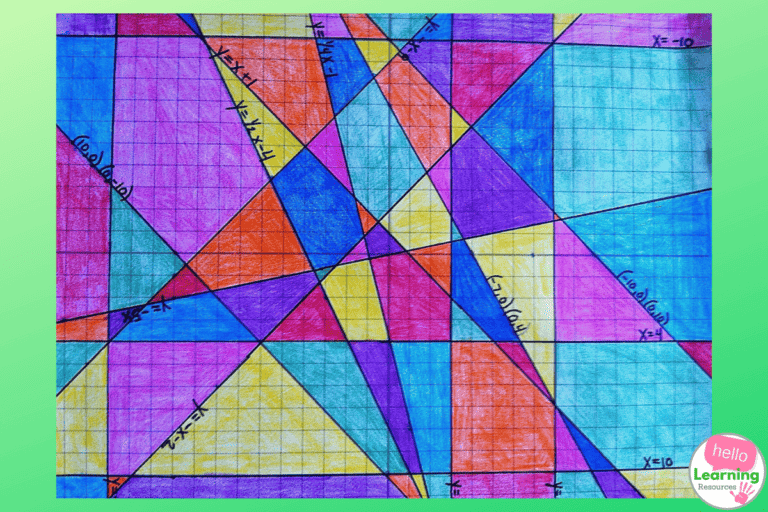Slope Stained Glass Window Project Hello LearningLinear Equations And Graphs Activities Paper And Pencil ActivitesStained Glasses Worksheets Teaching Resources Tpt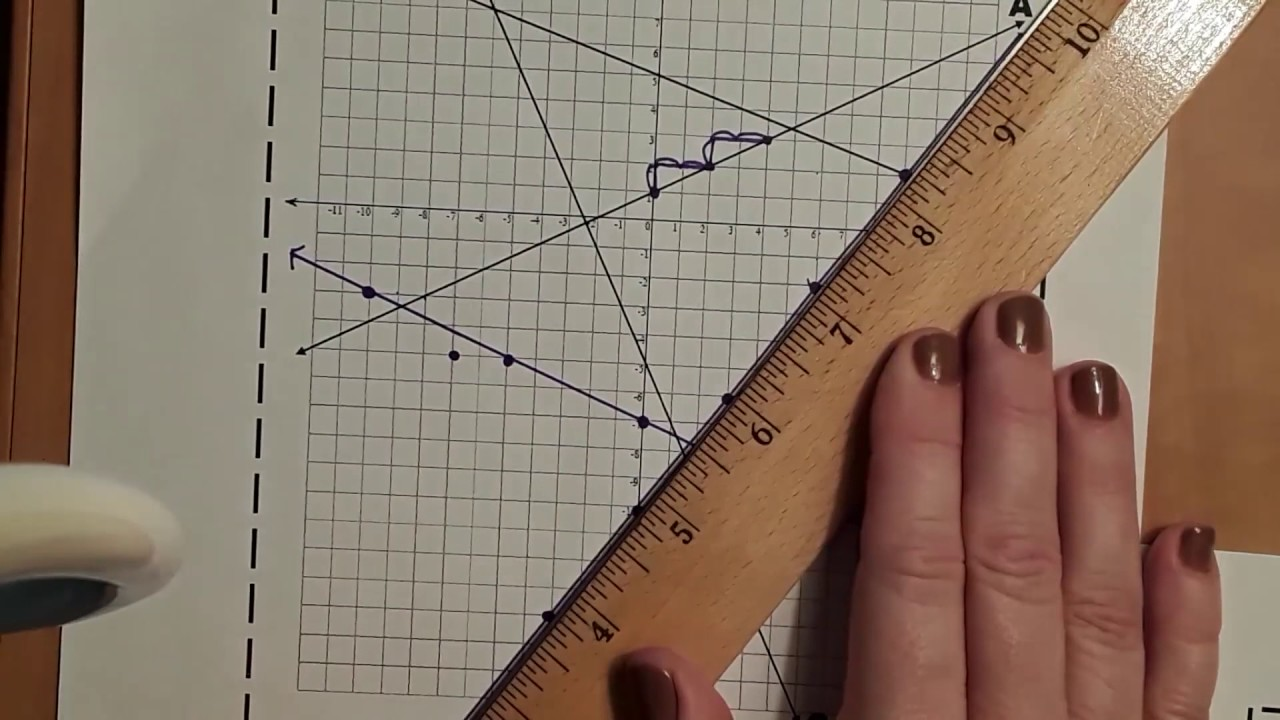Stained Glass YoutubeLinear Equation Stained Glass Window Project By Daniel Humble TptHow To Make Stained Glass With Pictures WikihowStained Glass Window Linear Equations Project Big Ideas Math Linear Equations Project Algebra Lessons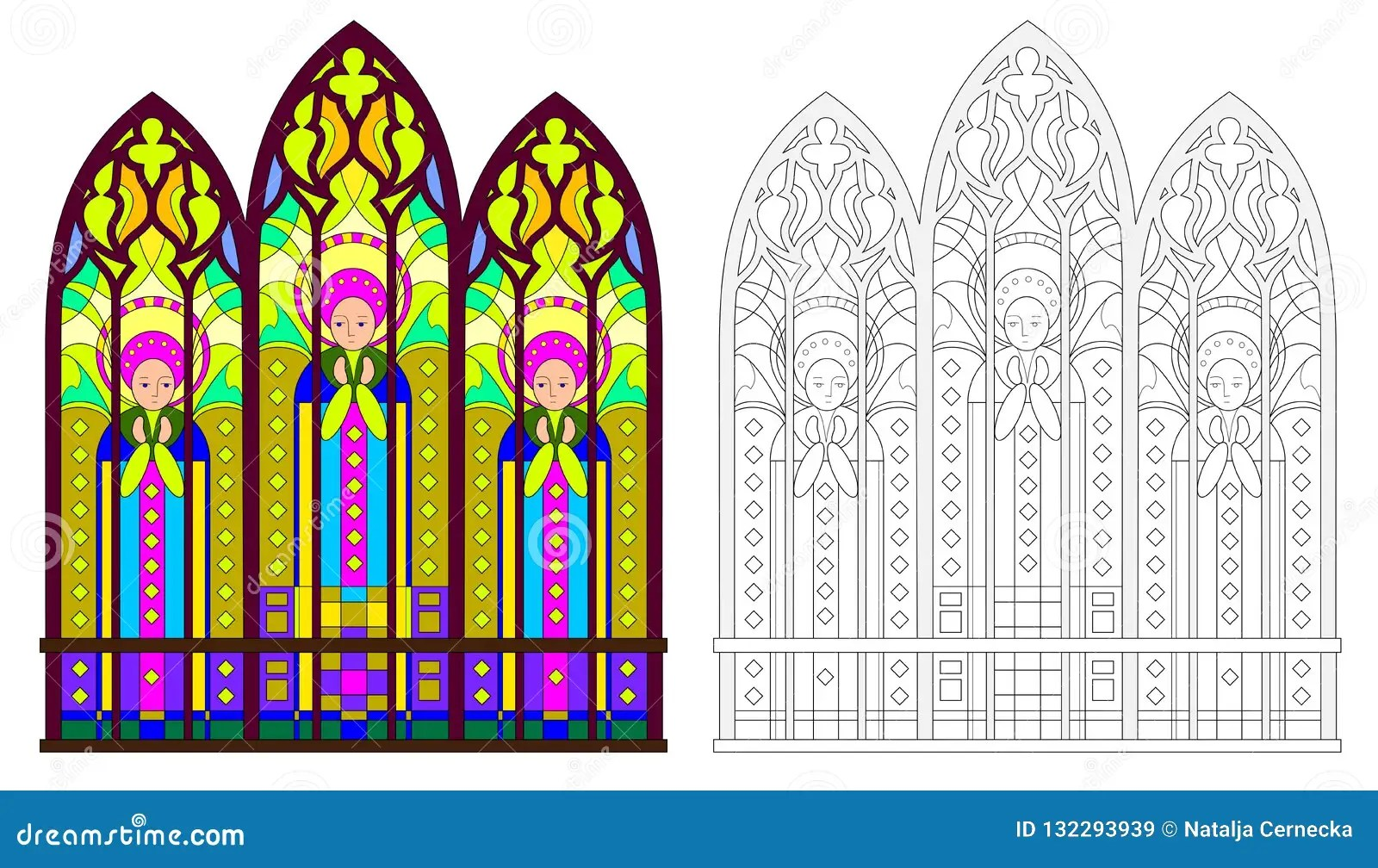Stained Glass Window Algebra Worksheet Printable Worksheets And Activities For Teachers Parents Tutors And Homeschool Families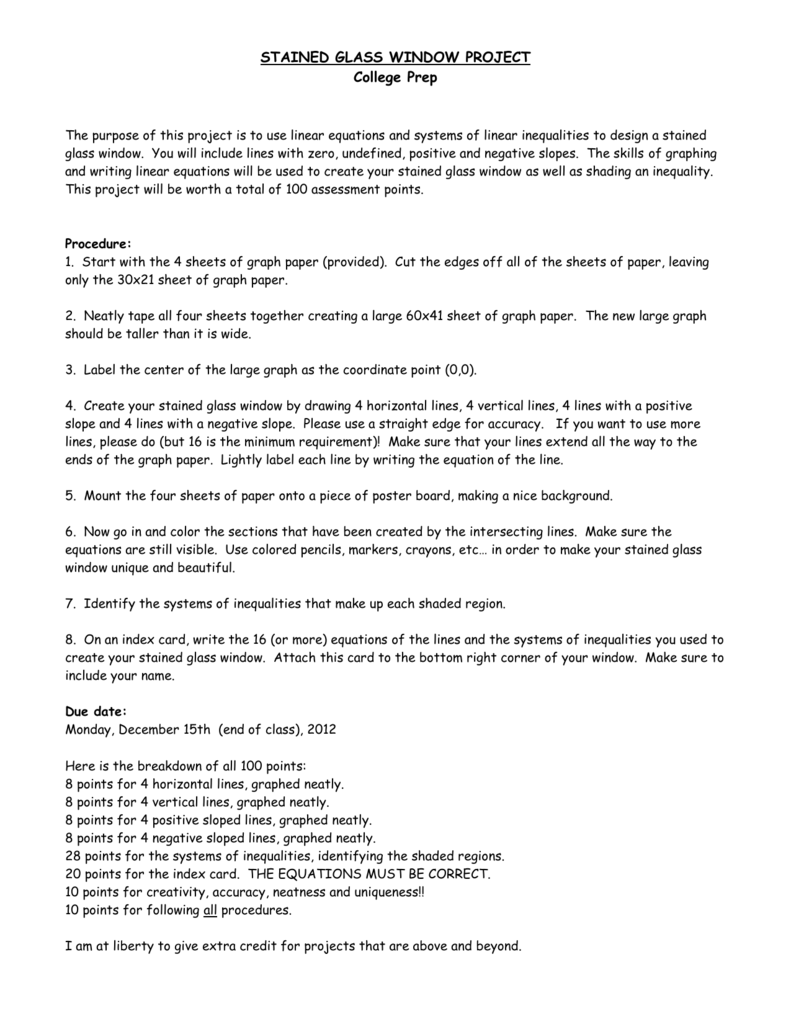Stained Glass Window Project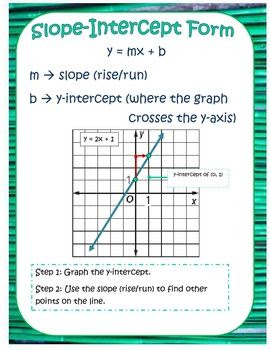Jmsmath8 Licensed For Non Commercial Use Only Graphing Linear Equations

Tags:stained glass window project graphing linear equations answerswindow

Sours: https://sacrifice4life.blogspot.com/2020/05/stained-glass-window-project-graphing.html
NTI Algebra Days 19-23 Part 1

How To Create Stained Glass By Graphing Linear Equations

Need an easy way to cover your windows while doubling as practice for your students? This is an easy way for students to practice graphing linear equations.

Students are given 18 equations written in slope-intercept form. All four types of slope are included(positive, negative, undefined, and zero). First, they will identify their slope and y-intercept of each equation. Then, they will graph the equations on the same coordinate plane. Students will then darken the lines and color however they like creating a picture with a stained glass effect.

The equations were created to force students to think about the slope of the line. An equation may be a positive slope but the y-intercept being near the top of the graph allows for students to think about how to graph points above and below the y-intercept. I ask students to graph at least two points above or below the y-intercept if the graph allows. This will show you which students have a true understanding of linear equations.

Sours: https://www.activityaftermath.com/blog-posts/stainedglassslope&copy; Digital Lesson.com Stained Glass Window Linear Equation Worksheet Circle three linear equations in each box and write them over the t-tables below. Complete each table with at least three ordered pairs (with coordinates of 10 or less) that are solutions to the linear equation. Then graph these twelve linear equations on the coordinate plane provided. Write the equation neatly on each line that you graph. When you are done graphing the equations use markers to color each section and create your stained glass window. x = -8 x = -5 x = -1 x=2 x=7 x=9 y = -9 y = -5 y = -2 y=1 y=6 y=8 y=x+5 y = 2x - 7 y = 4x + 8 y = 2x + 18 y=&frac14;x-6 y=&frac12;x-3 y = ___________ y = ___________ y = ___________ x y y = ___________ x y y = ___________ x y x y y = ___________ x y y = ___________ x y x y y = ___________ x y y = ___________ x y y = -x - 9 y = -2x + 8 y=-⅓x-3 y=-&frac14;x+5 y = -2x y = -x + 12 y = ___________ x y y = ___________ x y y = ___________ x y &copy; Digital Lesson.com Stained Glass Window Stained Glass Window Project On the coordinate plane below, graph the linear equations that you circled on the Linear Equations Worksheet. Use the three ordered pair solutions that you listed for each equation to graph it. Write the equation neatly on each line that you graph. When you are finished graphing the equations, use markers to color each section to create your stained glass window. 9 8 7 6 5 4 3 2 1 -9 -8 -7 -6 -5 -4 -3 -2 1 -1 2 3 4 5 6 7 8 9 -1 -2 -3 -4 -5 -6 -7 -8 -9 &copy; Digital Lesson.com Stained Glass Window Answer Key Sample Linear Equation Worksheet Circle three linear equations in each box and write them over the t-tables below. Complete each table with at least three ordered pairs (with coordinates of 10 or less) that are solutions to the linear equation. Then graph these twelve linear equations on the coordinate plane provided. Write the equation neatly on each line that you graph. When you are done graphing the equations use markers to color each section and create your stained glass window. (Underlined equations are used for sample project.) x = -8 x = -5 x = -1 x=2 x=7 x=9 y = -9 y = -5 y = -2 y=1 y=6 y=8 y=x+5 y = 2x - 7 y = 4x + 8 y = 2x + 18 y=&frac14;x-6 y=&frac12;x-3 y = -x - 9 y = -2x + 8 y=-⅓x-3 y=-&frac14;x+5 y = -2x y = -x + 12 x = -5 y = -9 y = 2x - 7 y = -2x + 8 x y x y x y x y -5 -5 -5 3 -2 -8 -2 5 -8 -9 -9 -9 0 -1 4 -7 -9 1 0 5 3 8 -2 2 x=2 y = -2 x y x y x y x y 2 2 2 5 -7 -1 3 -7 8 -2 -2 -2 -5 -10 -7 8 -2 4 4 0 -4 4 5 6 y = 2x + 18 y=-&frac14;x+5 x=9 y=6 x y x y x y x y 9 9 9 4 -3 8 -1 3 -5 6 6 6 4 8 0 -5 -4 -6 5 8 9 7 4 3 y=&frac14;x-6 y = -x + 12 &copy; Digital Lesson.com Stained Glass Window Answer Key Sample Stained Glass Window Project On the coordinate plane below, graph the linear equations that you circled on the Linear Equations Worksheet. Use the three ordered pair solutions that you listed for each equation to graph it. Write the equation neatly on each line that you graph. When you are finished graphing the equations, use markers to color each section to create your stained glass window. (This answer key goes with the sample Linear Equations Worksheet provided.) 9 y = 8 y = -&frac14; x+5 -x + 12 7 y=6 6 8 5 3 2 x=9 x=2 y= 2x x = -5 +1 4 1 -6 -5 -4 -3 -2 1 -1 2 3 4 5 6 7 8 9 -1 y = -2 -2 -3 -4 -7 -7 2x -8 y= -9 -5 -6 - 2x x-6 y= y=&frac14; +8 -7 -8 y = -9 -9 &copy; Digital Lesson.com Stained Glass Window Answer Key Stained Glass Window Project All 24 linear equations included in this project are graphed on this page. You may choose to have students graph all 24 equations, in which case you can make a transparency of this sheet and use it as an overlay to evaluate the work. (All 24 lines are included on this answer key.) 9 8 7 6 5 4 3 2 1 -9 -8 -7 -6 -5 -4 -3 -2 1 -1 2 3 4 5 6 7 8 9 -1 -2 -3 -4 -5 -6 -7 -8 -9 &copy; Digital Lesson.com Stained Glass Window Teacher Tips (1 of 2) Lesson Description: Stained Glass Window is a project that requires students to graph Linear Equations in order to create a colorful (yet mathematical) display window. Each student selects and graphs at least twelve linear equations from the equation bank to create their own unique window. This visual/ kinesthetic project will help students to clearly identify the equations of horizontal and vertical lines and to easily distinguish between positive and negative slope. Key vocabulary will also be developed. Math Content: Linear Equations, Graphing Linear Equations, Finding Solutions for Linear Equations, Slope, Y-Intercept, Coordinate Plane, Ordered Pairs, and Coordinates Time Required: 1-2 Class Periods Stained Glass Window includes: * 1 Stained Glass Window Linear Equations student worksheet * 1 Stained Glass Window Project student worksheet * 1 Stained Glass Window Project Linear Equations student worksheet sample * 1 Stained Glass Window Project Sample that goes with student worksheet sample * 1 Stained Glass Window Project Answer Key with all 24 equations graphed * 2 Stained Glass Window Teacher Tips pages * 1 Stained Glass Window Cover Sheet 8 pages in all! Materials Needed: Rulers, Colored Markers Suggested Grade Level: 5th - 8th Teacher Testimonial: Stained Glass Window is a project that provides needed practice for students in the area of Graphing Linear Equations. Students are able to be creative in selecting the equations that they want to graph and then choose colors in order to create their own unique Stained Glass Window. Then, they have the opportunity to put their window together with others in the class to create large Stained Glass Windows in the classroom. Teacher Tips: * The Stained Glass Window Project can be administered by the teacher in a number of ways: 1) Hand out the Linear Equations Worksheet and allow the students to choose the twelve linear equations that they will graph according to the worksheet directions. This will allow each student to have their own unique Stained Glass Window Project. 2) Prior to handing out the Linear Equations Worksheet, circle the twelve equations identified on the Sample Linear Equations Worksheet. By doing this, every student will end up with the exact same Stained Glass Window Project and you will already have a completed answer key (see the Sample Stained Glass Window Project). You can make a transparency of the Sample Stained Glass Window Project and place it over the student projects to quickly evaluate them. &copy; Digital Lesson.com Stained Glass Window Teacher Tips (2 of 2) Teacher Tips (continued) : 3) If you have multiple classes you may want to circle a different group of twelve equations to graph for each class. Then you can quickly create an answer key for each class I personally prefer to go with option # 1 and let each student create their own unique Stained Glass Window Project. It depends upon how you decide to evaluate the projects and whether or not you want a whole class to have the same final product. * It is also possible to have your students graph all 24 linear equations, but of course that would double the time involved in finding solutions and graphing the equations. You would need to give each student another Linear Equations Student Worksheet. An Answer Key showing the graphs of all 24 lines has been included and could be placed on a transparency in order to facilitate quick evaluation of the projects. * Each of the four boxes in the equation bank contains a group of similar linear equations. Discuss with student the equations for vertical lines, horizontal lines, positive slope, and negative slope. Teach students to recognize the equations that go with each graph type. * Make sure that students draw each line to the outer edge of the graph. * The teacher may want to evaluate the labeled lines that have been graphed prior to the color being added. After the windows are colored, it may be harder to see the equations. * To display the completed Stained Glass Window have the students write their names on the back of their windows, carefully cut them out, and then mount them to your classroom windows. The light coming through the projects will create a great window decoration. If you run out of window space you can always use bulletin boards. Try to center the display and use border paper around the windows if necessary. * Students that are familiar with the slope-intercept form of linear equations could also use the slope and y-intercept to graph (or to check) their lines. However, a few of the equations include a y-intercept that is not on this graph since the graphs only go up to ten. Copyright Notice: Rights are hereby granted for the purchaser of this lesson to use it within his/her classroom or home. Distribution to other teachers, schools, or parents is prohibited. All rights reserved by Digital Lesson.com. Middle School Math Treasures Newsletter: To receive DigitalLesson.com’s Middle School Math Treasures newsletter please visit our website at www.DigitalLesson.com and enter your e-mail address in the subscription box . You will then become eligible to receive new lesson updates, math resources and ideas, and a free printable math activity with each newsletter. You may unsubscribe at any time using the link in our newsletter. Enjoy your lesson!! Mark &copy; Digital Lesson.com
Sours: https://studylib.net/doc/25253012/stained-glass-project
Slope project

This past year I had a group of math students who really loved to be creative and especially loved to color!

So, when we were learning about slope in class I wanted some kind of creative project for my students to do where they could show their knowledge in a way that was more creative than a worksheet or quiz!

I searched around on the internet and Pinterest, and saw several versions of the slope stained glass window project, but none of them really fit the specific needs I had for my class.

So...I made my own version of this project so I could include everything I needed,  and it is now in my Teachers Pay Teachers store!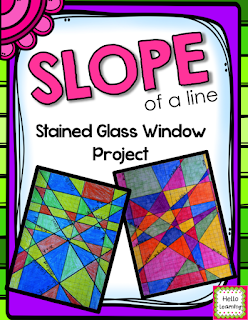In this project students had to choose several linear equations from a list and create a table of values that they plugged in to the equations to create x and y coordinates for each line.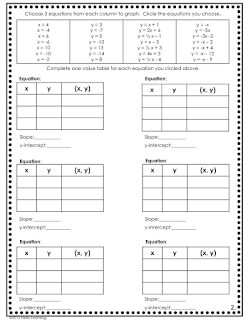They also determined the slope of each line, whether the slope was positive, negative, zero or undefined, and x and/or y intercepts.

Then they had to graph the lines on graph paper.  Each line needed to be labeled with the corresponding equation.

Bring on the colors!  I gave my students free range on what they used to add color to their design.  Crayons, colored pencils, markers, chalk....anything they wanted.  I encouraged them to put together a nice color scheme to make their final product visually appealing.

I created a 4 point rubric to grade the finished projects.  The kids had this rubric right away, so they knew exactly what they needed to do to get the highest grade.  I also a couple examples and had them use the rubric to determine a grade for each project.  I have found that giving the students a rubric and having them apply it to a sample project really helps them to see what high quality work looks like.

My class LOVED this project!  Here are some of their finished projects!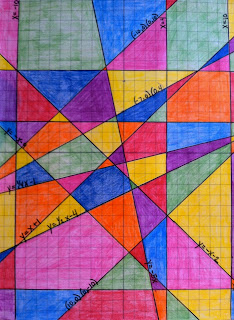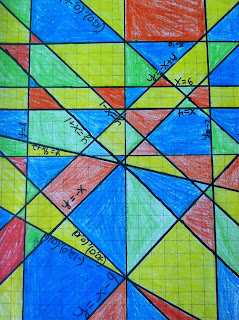I love doing projects with my math students!  Giving students another way to show what they know and actually apply the skills to a project (not just a worksheet) really hooks my students interest!

If you like this project, it is available in my Teachers Pay Teachers store!

Sours: http://hellolearning.blogspot.com/2015/08/slope-stained-glass-window-project.html

Stained Glass Blueprints Math Worksheet

A blank graph a copy of the stained glass blueprints ruler pencil. So make certain that you click the link make a replica of this pre filled google sheet for editing.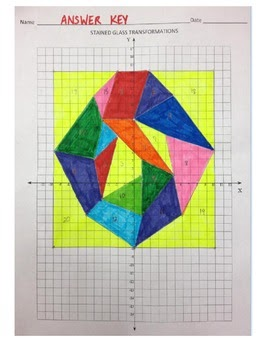Printables Of Stained Glass Worksheet Answer Key Geotwitter Kids

Showing top 8 worksheets in the category stained glass blueprints.Stained glass blueprints math worksheet. Students will each need the following materials. Each student selects and graphs at least twelve linear equations from the equation bank to create their own unique stained glass window. Showing top 8 worksheets in the category stained glass window.

All youve got to do when you arrive on their page is either select one of templates they give or start fresh. Worksheets are digital lesson stained glass blue s stained glass blue s lesson plan title stained glass grade level 3 4 your digital lesson accelerated stained glass linear equations project graphing linear equations graphing lines finding equations of lines. When completed the correctly graphed lines will created a stained glass window which can be colored for a fun art math crossover project.

So that we tried to identify some great 10 stained glass blueprints math worksheet answers graphic to suit your needs. Some of the worksheets for this concept are digital lesson stained glass blue s stained glass blue s lesson plan title stained glass grade level 3 4 your digital lesson accelerated stained glass linear equations project graphing linear equations graphing lines finding equations of lines. Some of the worksheets for this concept are digital lesson stained glass blue s stained glass blue s lesson plan title stained glass grade level 3 4 your digital lesson accelerated stained glass linear equations project graphing linear equations graphing lines finding equations of.

Seriously we also have been remarked that 10 stained glass blueprints math worksheet answers is being one of the most popular issue referring to document example at this moment. Displaying all worksheets related to stained glass blueprints. Some of the worksheets displayed are digital lesson stained glass blue s stained glass blue s lesson plan title stained glass grade level 3 4 your digital lesson accelerated stained glass linear equations project graphing linear equations graphing lines finding equations of lines.

Stained glass window graphing project stained glass window is a project that requires students to graph linear equations in order to create a colorful yet mathematical display window. Some of the worksheets displayed are digital lesson lesson 2 telling stories through stained glass stage 3 stained glass windows stained glass window lesson plan title stained glass grade level 3 4 your digital lesson stained glass windows graphing linear equations. Stained glass blueprints displaying top 8 worksheets found for this concept.

Zero and undefined slopes. In this article we have 20 great pics with regard to stained glass blueprints math worksheetwe expect you enjoyed it and if you wish to download the photo in high quality click the image and you will be redirected to the download page of stained glass blueprints math worksheet. Displaying top 8 worksheets found for stained glass blueprints.Free Worksheets For Graphing Linear Equations Finding The Slope40 Stained Glass Window Project Algebra 2 Visual Art Scoop8th Grade Ms Taimanglo S Math ClassStained Glass BlueprintsSlope Intersept Form Math Joom ClubStained Glass Windows Worksheet The Best Worksheets Image CollectionStain Glass Math Worksheets Teaching Resources TptStained Glass Blueprints Math Worksheet Stained Glass GraphingStained Glass Blueprints Math Worksheet Answers 94 Best StainedStained Glass Blueprints Math Worksheet BriefencountersLinear Equations Stained Glass Worksheets Teaching Resources TptPinterestMath Resources K12 Supplementary Collections 8760595684 StainedPatterns And Expressions 5th Grade WorksheetsStained Glass Geometry Crayola ComStained Glass GraphingStained Glass Worksheets The Best Worksheets Image CollectionStained Glass Blueprints Math Worksheet Answers Fun Printable144 Best Mosaic Images On Pinterest Stained Glass Blueprints MathPercent Discount Taxes And Tips Coloring Worksheet By Sales TaxColor By Number Mosaic Math Coloring Mosaics And WorksheetsStained Glass Blueprints Math Worksheet Answer Key Best StainedStained Glass Window Project Algebra 2 Glasses BlogColor By Number For Kids Printouts Mosaic Math Worksheets Printable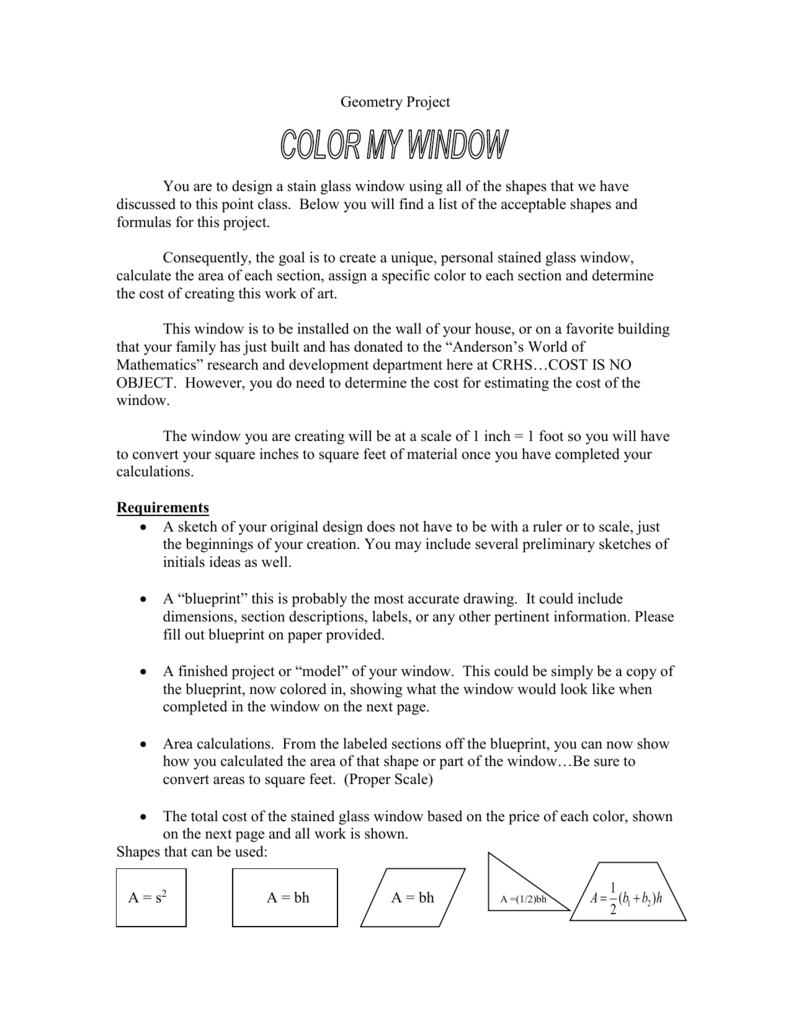Geometry ProjectStained Glass Math Project Answer KeyStain Glass Math Worksheets Teaching Resources Tpt8 Best Blueprints Images On Pinterest Stained Glass Blueprints MathStained Glass GraphingStain Glass Slope Worksheets Teaching Resources TptStained Glass Window Graphing Linear Functions By Emily Comer Tpt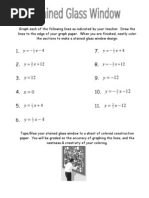Stained Glass Window Graphing Linear EquationsStained Glass Math Activity Linear Equations ProjectConstruction Math Worksheets Grade Coloring Preschool Free

Sours: https://ivuyteq.blogspot.com/2019/11/stained-glass-blueprints-math-worksheet.html?m=1

171 172 173 174 175